# Convergence of vertex-reinforced jump processes to an extension of the supersymmetric hyperbolic nonlinear sigma model

## Abstract

In this paper, we define an extension of the supersymmetric hyperbolic nonlinear sigma model introduced by Zirnbauer. We show that it arises as a weak joint limit of a time-changed version introduced by Sabot and Tarrès of the vertex-reinforced jump process. It describes the asymptotics of rescaled crossing numbers, rescaled fluctuations of local times, asymptotic local times on a logarithmic scale, endpoints of paths, and last exit trees.

This is a preview of subscription content, log in to check access.

## Change history

• ### 26 February 2020

In the original publication, “Acknowledgements” section was incorrect. The correct “Acknowledgements” is given below.

## References

1. 1.

Angel, O., Crawford, N., Kozma, G.: Localization for linearly edge reinforced random walks. Duke Math. J. 163(5), 889–921 (2014). https://doi.org/10.1215/00127094-2644357

2. 2.

Basdevant, A.L., Singh, A.: Continuous-time vertex reinforced jump processes on Galton–Watson trees. Ann. Appl. Probab. 22(4), 1728–1743 (2012). https://doi.org/10.1214/11-AAP811

3. 3.

Collevecchio, A.: On the transience of processes defined on Galton–Watson trees. Ann. Probab. 34(3), 870–878 (2006). https://doi.org/10.1214/009117905000000837

4. 4.

Collevecchio, A.: Limit theorems for vertex-reinforced jump processes on regular trees. Electron. J. Probab. 14(66), 1936–1962 (2009). https://doi.org/10.1214/EJP.v14-693

5. 5.

Davis, B., Volkov, S.: Continuous time vertex-reinforced jump processes. Probab. Theory Relat. Fields 123(2), 281–300 (2002). https://doi.org/10.1007/s004400100189

6. 6.

Davis, B., Volkov, S.: Vertex-reinforced jump processes on trees and finite graphs. Probab. Theory Relat. Fields 128(1), 42–62 (2004). https://doi.org/10.1007/s00440-003-0286-y

7. 7.

Disertori, M., Merkl, F., Rolles, S.: Localization for a nonlinear sigma model in a strip related to vertex reinforced jump processes. Commun. Math. Phys. 332(2), 783–825 (2014). https://doi.org/10.1007/s00220-014-2102-1

8. 8.

Disertori, M., Merkl, F., Rolles, S.: A comparison of a nonlinear sigma model with general pinning and pinning at one point. Electron. J. Probab. 21, 27, 16 (2016). https://doi.org/10.1214/16-EJP4340

9. 9.

Disertori, M., Sabot, C., Tarrès, P.: Transience of edge-reinforced random walk. Commun. Math. Phys. 339(1), 121–148 (2015). https://doi.org/10.1007/s00220-015-2392-y

10. 10.

Disertori, M., Spencer, T.: Anderson localization for a supersymmetric sigma model. Commun. Math. Phys. 300(3), 659–671 (2010). https://doi.org/10.1007/s00220-010-1124-6

11. 11.

Disertori, M., Spencer, T., Zirnbauer, M.: Quasi-diffusion in a 3D supersymmetric hyperbolic sigma model. Commun. Math. Phys. 300(2), 435–486 (2010). https://doi.org/10.1007/s00220-010-1117-5

12. 12.

Keane, M., Rolles, S.: Edge-reinforced random walk on finite graphs. In: Infinite Dimensional Stochastic Analysis (Amsterdam, 1999), pp. 217–234. Royal Netherlands Academy of Arts and Sciences, Amsterdam (2000)

13. 13.

Merkl, F., Rolles, S.: Linearly edge-reinforced random walks. In: Dynamics and Stochastics. Festschrift in Honor of M. S. Keane. Selected Papers Based on the Presentations at the Conference ‘Dynamical Systems, Probability Theory, and Statistical Mechanics’, Eindhoven, The Netherlands, January 3–7, 2005, on the Occasion of the 65th Birthday of Mike S. Keane., pp. 66–77. IMS, Institute of Mathematical Statistics, Beachwood, OH (2006). https://doi.org/10.1214/074921706000000103

14. 14.

Sabot, C., Tarrès, P.: Edge-reinforced random walk, vertex-reinforced jump process and the supersymmetric hyperbolic sigma model. JEMS 17(9), 2353–2378 (2015)

15. 15.

Sabot, C., Tarrès, P.: Inverting Ray-Knight identity. Probab. Theory Relat. Fields 165(3–4), 559–580 (2016). https://doi.org/10.1007/s00440-015-0640-x

16. 16.

Sabot, C., Tarrès, P., Zeng, X.: The vertex reinforced jump process and a random Schrödinger operator on finite graphs. Ann. Probab. 45(6A), 3967–3986 (2017). https://doi.org/10.1214/16-AOP1155

17. 17.

Sabot, C., Zeng, X.: A random Schrödinger operator associated with the vertex reinforced jump process and the edge reinforced random walk. Preprint arXiv:1507.07944 (2015)

18. 18.

Tarrès, P.: Localization of reinforced random walks. Preprint arXiv:1103.5536 (2011)

19. 19.

Zeng, X.: How vertex reinforced jump process arises naturally. Ann. Inst. Henri Poincaré Probab. Stat. 52(3), 1061–1075 (2016). https://doi.org/10.1214/15-AIHP671

20. 20.

Zirnbauer, M.: Fourier analysis on a hyperbolic supermanifold with constant curvature. Commun. Math. Phys. 141(3), 503–522 (1991). http://projecteuclid.org/getRecord?id=euclid.cmp/1104248390

## Acknowledgements

The authors would like to thank an anonymous referee and the associate editor for very constructive comments helping us to improve the paper.

## Author information

Authors

### Corresponding author

Correspondence to Silke W. W. Rolles.

## Appendices

### Proof of Lemma 1

We abbreviate

\begin{aligned} \nabla v=v_{i_0}-v_{i_1}=-v_{i_1}. \end{aligned}
(124)

In the whole proof, we work only on the events $$B_\sigma (M)$$. All Landau symbols O are understood in the limit as $$\sigma \rightarrow \infty$$. Note that on $$B_\sigma (M)$$, we have $$k_{ij}\rightarrow \infty$$ for anyas $$\sigma \rightarrow \infty$$, with $$k_{ij}/\sigma$$ being bounded away from 0. Hence, by Stirling’s formula,

\begin{aligned} k_{ij}!=\sqrt{2\pi }e^{-k_{ij}}k_{ij}^{k_{ij}+\frac{1}{2}}\left( 1+O_M\left( \sigma ^{-1}\right) \right) . \end{aligned}
(125)

For, one has

\begin{aligned} k_{ij}\left( \frac{W_{ij}l_i}{2}\right) ^{k_{ij}}\frac{1}{k_{ij}!} = \sqrt{\frac{k_{ij}}{2\pi }} \left( \frac{W_{ij}l_ie}{2k_{ij}}\right) ^{k_{ij}} \left( 1+O_M\left( \sigma ^{-1}\right) \right) . \end{aligned}
(126)

If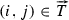, this is the $$k_{ij}$$-dependent part in the definition (23) of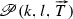. Using

\begin{aligned} k_{ij}=l_{i_0}\left( \omega _{ij}+l_{i_0}^{-1/2}\kappa _{ij}\right) = l_{i_0}\omega _{ij} \left( 1+O_{M,W}\left( \sigma ^{-1/2}\right) \right) \end{aligned}
(127)

and the symmetry $$\omega _{ij}=\omega _{ji}$$, we deduce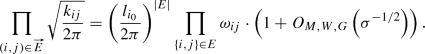(128)

One has

\begin{aligned} \frac{W_{ij}l_ie}{2k_{ij}} =\frac{W_{ij}l_{i_0} e^{1+2v_i}}{2(l_{i_0}\omega _{ij}+l_{i_0}^{1/2}\kappa _{ij})} =\frac{\omega _{ij}e^{1+v_i-v_j}}{\omega _{ij}+l_{i_0}^{-1/2}\kappa _{ij}}. \end{aligned}
(129)

Consequently, we obtain

\begin{aligned} \log \left[ \left( \frac{W_{ij}l_ie}{2k_{ij}}\right) ^{k_{ij}}\right] =&\,\, l_{i_0}\left( \omega _{ij}+l_{i_0}^{-1/2}\kappa _{ij}\right) \left( \log \omega _{ij}+1+v_i-v_j\right) \nonumber \\&-l_{i_0}\left( \omega _{ij}+l_{i_0}^{-1/2}\kappa _{ij}\right) \log \left( \omega _{ij}+l_{i_0}^{-1/2}\kappa _{ij}\right) . \end{aligned}
(130)

Using the Taylor expansion $$x\log x= x_0\log x_0+(1+\log x_0)(x-x_0)+\frac{(x-x_0)^2}{2x_0}+O((x-x_0)^3)$$ as $$x\rightarrow x_0$$ at $$x_0=\omega _{ij}$$ for the second term, we deduce

\begin{aligned} \log \left[ \left( \frac{W_{ij}l_ie}{2k_{ij}}\right) ^{k_{ij}}\right] =&\,\, l_{i_0}\left( \omega _{ij}+l_{i_0}^{-1/2}\kappa _{ij}\right) (\log \omega _{ij}+1+v_i-v_j)\nonumber \\&-l_{i_0}\left( \omega _{ij}\log \omega _{ij}+(1+\log \omega _{ij})l_{i_0}^{-1/2}\kappa _{ij} +\frac{\kappa _{ij}^2}{2\omega _{ij}l_{i_0}} +O_M\left( \sigma ^{-3/2}\right) \right) \nonumber \\ =&\,\, l_{i_0}\omega _{ij}(1+v_i-v_j)+\sqrt{l_{i_0}}\kappa _{ij}(v_i-v_j) -\frac{\kappa _{ij}^2}{2\omega _{ij}} +O_M\left( \sigma ^{-1/2}\right) . \end{aligned}
(131)

Since $$\omega _{ij}=\omega _{ji}$$ and withthere isas well, we have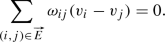(132)

Note that(133)

Using this, Kirchhoff’s rule (20) for $$k_{ij}$$, and the definition (124) of $$\nabla v$$, we deduce(134)

Combining (132) and (134) with (131) yields(135)

Inserting (128) and (135) into (126) and using the definition (23) of $$\mathscr {P}$$ yields(136)

Using (127) and the fact thatis a spanning tree directed towards $$i_1$$, we obtain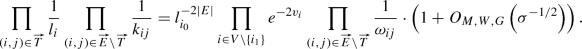(137)

Combining this with (136) yields(138)

Using $$\nabla v=-v_{i_1}$$, the claim follows. $$\square$$

### B Proof of Lemma 4: Gaussian integral over currents

We endow every undirected edge in E with a counting direction. For $$\kappa \in \mathscr {H}$$ and $$i,j\in V$$ such that $$\{i,j\}\in E$$, we introduce the following variables

\begin{aligned} I_{ij}=\frac{1}{\sqrt{2}}(\kappa _{ij}-\kappa _{ji}), \quad J_{ij}=\frac{1}{\sqrt{2}}(\kappa _{ij}+\kappa _{ji}). \end{aligned}
(139)

Note that I is antisymmetric ($$I_{ij}=-I_{ji}$$) and J is symmetric ($$J_{ij}=J_{ji}$$). Recall that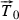denotes a directed reference spanning tree and $$T_0$$ its undirected version. Recall that the restriction mapis an isomorphism. In other words, the components $$\kappa _{\alpha \beta }$$,, of $$\kappa \in \mathscr {H}$$ can be chosen arbitrarily while all other $$\kappa _{ij}$$,, are determined by the first. We define now a linear map. Given, we set $$\kappa =\iota ^{-1}(\tilde{\kappa })$$ and

\begin{aligned} L(\tilde{\kappa }) =\left( (I_{ij}(\kappa ))_{\{i,j\}\in E\setminus T_0},(J_{ij}(\kappa ))_{\{i,j\}\in E} \right) , \end{aligned}
(140)

where the vertices i and j in $$I_{ij}$$ are ordered with respect to the counting direction of the edge $$\{i,j\}$$, in order to have no ambiguity with the sign of $$I_{ij}$$. We claim that the determinant of L equals $$\pm 2^{\frac{|V|-1}{2}}$$. In other words, this yields the change of measure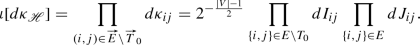(141)

Indeed, with an appropriate order of indices, the matrix associated to L is given by(142)

which can be written as follows, by an appropriate choice of order on the second index:(143)

We order the indices $$(\alpha ,\beta )$$ with $$\{\alpha ,\beta \}\in E\setminus T_0$$ in the second block column successively by groups of two, associated to each nonoriented edge $$\{\alpha ,\beta \}\in E\setminus T_0$$, taking first the oriented edge corresponding to the arbitrary counting direction. We claim that the Jacobian matrix above takes the following block triangular form:

\begin{aligned} \left( \begin{array}{cc} \sqrt{2}\, {\text {id}}_{|T_0|\times |T_0|}&{} \left( *\right) _{|T_0|\times 2|E\setminus T_0|}\quad \\ (0)_{2|E\setminus T_0|\times |T_0|} &{}\dfrac{1}{\sqrt{2}} \left( \begin{array}{cc} 1 &{}-1\\ 1 &{} 1 \end{array} \right) \otimes {\text {id}}_{|E\setminus T_0|\times |E\setminus T_0|} \end{array} \right) , \end{aligned}
(144)

In order to see why the first block column takes the claimed form, letand consider. Then, $$\kappa =\iota ^{-1}(\tilde{\kappa })$$ is given by $$\kappa _{\alpha \beta }=\kappa _{\beta \alpha }=1$$ and $$\kappa _{ij}=0$$ otherwise. This implies $$I_{ij}(\kappa )=0$$ for all $$\{i,j\}\in E$$, $$J_{\alpha \beta }(\kappa )=\sqrt{2}$$, and $$J_{ij}(\kappa )=0$$ otherwise. This explains the blocks $$\sqrt{2}{\text {id}}$$ and 0. The expression for the lower right block in the matrix (144) follows from the definition (139) using that $$\kappa _{\alpha \beta }$$ withare linearly independent variables. We conclude

\begin{aligned} |\det L|=\sqrt{2}^{|T_0|}\left[ \det \dfrac{1}{\sqrt{2}} \left( \begin{array}{cc} 1 &{}-1\\ 1 &{} 1 \end{array} \right) \right] ^{|E\setminus T_0|} =2^{\frac{|T_0|}{2}}\cdot 1^{|E\setminus T_0|} =2^{\frac{|V|-1}{2}} , \end{aligned}
(145)

in other words (141) holds.

Note that for all $$\{i,j\}\in E$$,

\begin{aligned} I_{ij}^2+J_{ij}^2 =\frac{1}{2}\left( (\kappa _{ij}-\kappa _{ji})^2+(\kappa _{ij}+\kappa _{ji})^2\right) =\kappa _{ij}^2+\kappa _{ji}^2. \end{aligned}
(146)

Consequently, we obtain(147)

For $$e\in E{\setminus } T_0$$, let $$c_e$$ be the unique oriented cycle in $$T_0\cup \{e\}$$ containing the edge e in its counting direction. For another edge $$g\in E$$, let $$\sigma _{eg}$$ be $$+1$$ if e and g appear in the same counting direction in $$c_e$$, $$-1$$ if e and g appear in opposite counting directions in $$c_e$$, and 0 if $$g\notin c_e$$. We define the matrix $$B=(B_{ef})_{e,f\in E\setminus T_0}$$ by

\begin{aligned} B_{ee}=\sum _{g\in c_e}\frac{1}{\omega _g}, \quad B_{ef}=\sum _{g\in c_e\cap c_f} \frac{\sigma _{eg}\sigma _{fg}}{\omega _g}\quad \text {for }e\ne f. \end{aligned}
(148)

Let $$I=(I_{ij})_{\{i,j\}\in E\setminus T_0}$$ denote the restriction to $$E\setminus T_0$$. Note that the full vector $$(I_{ij})_{\{i,j\}\in E}$$ can be retrieved from its restriction I using the formula $$I_f=\sum _{e\in E\setminus T_0}\sigma _{ef}I_e$$. This formula implies

\begin{aligned} \sum _{\{i,j\}\in E}\frac{I_{ij}^2}{2\omega _{ij}} =\sum _{e\in E\setminus T_0}\sum _{f\in E\setminus T_0}I_eI_f \sum _{g\in E}\frac{\sigma _{eg}\sigma _{fg}}{2\omega _g} = \frac{1}{2} I^tBI. \end{aligned}
(149)

We abbreviate $$dI=\prod _{\{i,j\}\in E\setminus T_0}dI_{ij}$$. Using the formula for the determinant of B given as last displayed formula on page 20 of , which is based on the matrix-tree theorem, we obtain

\begin{aligned}&\int _{{\mathbb {R}}^{E\setminus T_0}}\prod _{\{i,j\}\in E} \exp \left( -\frac{I_{ij}^2}{2\omega _{ij}}\right) \prod _{\{i,j\}\in E\setminus T_0}dI_{ij} = \int _{{\mathbb {R}}^{E\setminus T_0}}e^{-\frac{1}{2}I^tBI}dI \nonumber \\&\quad = \frac{(2\pi )^{\frac{|E|-|V|+1}{2}}}{\sqrt{\det B}} = (2\pi )^{\frac{|E|-|V|+1}{2}}\frac{\prod _{\{i,j\}\in E}\sqrt{\omega _{ij}}}{ \sqrt{\sum _{S\in {\mathscr {T}}}\prod _{\{i,j\}\in S}\omega _{ij}}}. \end{aligned}
(150)

We conclude that(151)

### Fact 1

\begin{aligned}&\int _{\varOmega _{i_0}} \exp \left( \sum _{\{i,j\}\in E} W_{ij}\left( 1-\cosh (v_i-v_j)\right) \right) \\&\quad \cdot \sqrt{\sum _{S\in {\mathscr {T}}}\prod _{\{i,j\}\in S}W_{ij}e^{v_i+v_j}}\prod _{i\in V\setminus \{i_0\}} \frac{e^{-v_i}}{\sqrt{2\pi }}\,dv_i =1. \end{aligned}

We refer to formula (3) in Theorem 2 in  with $$\phi _i=1$$ for all i, which gives a new proof of this normalization using an interpretation of u through the Green’s function of random Schrödinger operators.

The first proof of Fact 1 was given in . It heavily uses the supersymmetry of the model, which is only seen in the version of the $${H^{2|2}}$$ model with Grassmann variables, cf. formula (5.1) and Proposition 2 in Appendix C of . Note that the reference point $$i_0$$ is not mentioned explicitly in , but the pinning strengths $$\varepsilon _i$$ in that paper play the role of the weights $$W_{ii_0}$$ connecting any vertex $$i\in V{\setminus }\{i_0\}$$ to the reference vertex $$i_0$$, while the coupling constants $$\beta J_{ij}$$ play the role of all other weights $$W_{ij}$$, $$\{i,j\}\in E$$ with $$i,j\ne i_0$$.

The link between the $${H^{2|2}}$$ model in horospherical coordinates, treated in , and its tree version, used in the current paper, is given by the matrix tree theorem stated in formula (2.17) in . More precisely, the sum $$\sum _{T'\in {\mathscr {T}}}\prod _{\{i,j\}\in T'}W_{ij}e^{u_i+u_j}$$ arises from the matrix tree theorem as the same determinant that occurs when integrating out the Grassmann variables $$\overline{\psi }_i$$ and $$\psi _i$$. A variant of this argument concerning only the marginal of u with respect to $$\mu _{i_0}^\mathrm {susy}$$ is also described in ; see formula (1.4) in that paper for the statement.

There is at least another proof of Fact 1 that does not use supersymmetry. In the paper  of Sabot and Tarrès, the normalization comes from the fact that the marginal in u of the measure is interpreted as a probability distribution for a random variable associated to asymptotic behavior of the vertex-reinforced jump process.

### C2 Density of paths

According to the first displayed formula on page 569 of , the probability that, at time t, the process Z has followed a path $$Z_0=\pi _ 0$$, $$\pi _1$$, $$\ldots$$, $$Z_t = \pi _n$$ with jump times respectively in $$[t_i,t_i+dt_i]$$, $$i=1,\ldots ,n$$, where $$t_0=0<t_1<\cdots< t_n < t =t_{n+1}$$, is given by

\begin{aligned} \exp \left( \sum _{\{i,j\}\in E} W_{ij}\left( 1-\sqrt{1+2\ell _i}\sqrt{1+2\ell _j}\right) \right) \prod _{i\in V\setminus \{i_1\}}\frac{1}{\sqrt{1+2\ell _i}} \prod _{i=1}^n W_{\pi _{i-1}\pi _j}dt_i. \end{aligned}
(152)

This formula is precisely the cited formula in the special case of offset $$\varphi =1$$, with the abbreviations from  already inserted. Their time t and local times $$\ell _i$$ differ from our time $$\sigma =2t$$ and local times $$l_i=2\ell _i$$ by a factor of 1 / 2 as can be seen by comparing formula (13) with the expression for D(s) on page 567 of . Rescaling all times by this factor 1 / 2 yields that the expression in (152) equals

\begin{aligned} \exp \left( \sum _{\{i,j\}\in E} W_{ij}\left( 1-\sqrt{1+l_i}\sqrt{1+l_j}\right) \right) \prod _{i\in V\setminus \{i_1\}}\frac{1}{\sqrt{1+l_i}} \prod _{i=1}^n \frac{W_{\pi _{i-1}\pi _j}}{2}d\sigma _i. \nonumber \\ \end{aligned}
(153)

Formula (51) is just obtained from this density by integrating over an event. Formula (153) in almost the same notation is also cited in formula (2) in Section 3.3 of .

### Fact 2

For all $$i_0,i_1\in V$$, $$k\in \mathscr {K}^+_{i_0,i_1}$$, and all, one has(154)

with $$k_i$$ defined in (53).

In Lemma 6 of , the first equality in (154) is stated with an additional summation over all. However, the proof of this lemma is based on a combinatorial lemma (Lemma 5 of ) which is also applicable for any fixed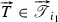, without summation over. The idea behind this combinatorial lemma is the following: we attach to every vertex $$i\in V$$ a sequence of length $$k_i$$ consisting of directed edges (ij) such that vertex $$j\in V$$ occurs precisely $$k_{ij}$$ times and the union of the last edges from all $$i\ne i_1$$ yields. Starting at $$i_0$$ we construct a path by always traversing the first edge which was not used earlier at the current location. This yields that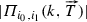equals the following product over multinomial coefficients, counting the number of choices at every vertex $$i\in V$$: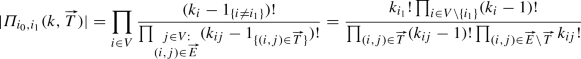(155)

## Rights and permissions

Reprints and Permissions